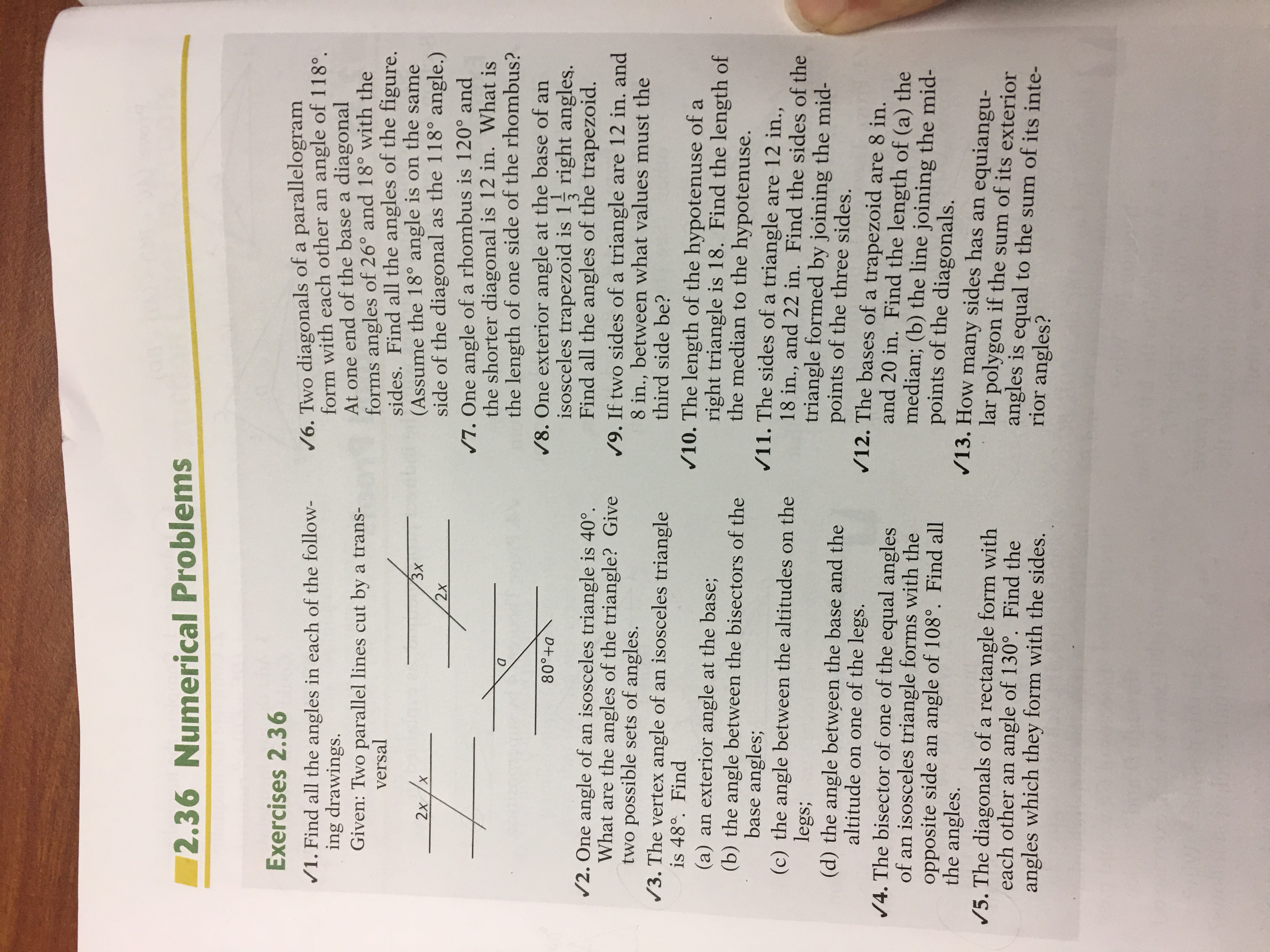# 2.36 Numerical ProblemsExercises 2.36/6. Two diagonals of a parallelogramform with each other an angle of 118°.At one end of the base a diagonalforms angles of 26° and 18° with thesides. Find all the angles of the figure.(Assume the 18° angle is on the sameside of the diagonal as the 118° angle.)/7. One angle of a rhombus is 120° andthe shorter diagonal is 12 in. What isthe length of one side of the rhombus?/8. One exterior angle at the base of anisosceles trapezoid is 1 right angles.Find all the angles of the trapezoid./9. If two sides of a triangle are 12 in. and8 in., between what values must thethird side be?/1. Find all the angles in each of the follow-ing drawings.Given: Two parallel lines cut by a trans-versal3х2хX.2x80°+a/2. One angle of an isosceles triangle is 40°.What are the angles of the triangle? Givetwo possible sets of angles./3. The vertex angle of an isosceles triangleis 48°. Find(a) an exterior angle at the base;(b) the angle between the bisectors of thebase angles;(c) the angle between the altitudes on thelegs;(d) the angle between the base and thealtitude on one of the legs./4. The bisector of one of the equal anglesof an isosceles triangle forms with theopposite side an angle of 108°. Find allthe angles./5. The diagonals of a rectangle form witheach other an angle of 130°. Find theangles which they form with the sides./10. The length of the hypotenuse of aright triangle is 18. Find the length ofthe median to the hypotenuse./11. The sides of a triangle are 12 in.,18 in., and 22 in. Find the sides of thetriangle formed by joining the mid-points of the three sides./12. The bases of a trapezoid are 8 in.and 20 in. Find the length of (a) themedian; (b) the line joining the mid-points of the diagonals./13. How many sides has an equiangu-lar polygon if the sum of its exteriorangles is equal to the sum of its inte-rior angles?

Question
2 viewshelp_outlineImage Transcriptionclose2.36 Numerical Problems Exercises 2.36 /6. Two diagonals of a parallelogram form with each other an angle of 118°. At one end of the base a diagonal forms angles of 26° and 18° with the sides. Find all the angles of the figure. (Assume the 18° angle is on the same side of the diagonal as the 118° angle.) /7. One angle of a rhombus is 120° and the shorter diagonal is 12 in. What is the length of one side of the rhombus? /8. One exterior angle at the base of an isosceles trapezoid is 1 right angles. Find all the angles of the trapezoid. /9. If two sides of a triangle are 12 in. and 8 in., between what values must the third side be? /1. Find all the angles in each of the follow- ing drawings. Given: Two parallel lines cut by a trans- versal 3х 2х X. 2x 80°+a /2. One angle of an isosceles triangle is 40°. What are the angles of the triangle? Give two possible sets of angles. /3. The vertex angle of an isosceles triangle is 48°. Find (a) an exterior angle at the base; (b) the angle between the bisectors of the base angles; (c) the angle between the altitudes on the legs; (d) the angle between the base and the altitude on one of the legs. /4. The bisector of one of the equal angles of an isosceles triangle forms with the opposite side an angle of 108°. Find all the angles. /5. The diagonals of a rectangle form with each other an angle of 130°. Find the angles which they form with the sides. /10. The length of the hypotenuse of a right triangle is 18. Find the length of the median to the hypotenuse. /11. The sides of a triangle are 12 in., 18 in., and 22 in. Find the sides of the triangle formed by joining the mid- points of the three sides. /12. The bases of a trapezoid are 8 in. and 20 in. Find the length of (a) the median; (b) the line joining the mid- points of the diagonals. /13. How many sides has an equiangu- lar polygon if the sum of its exterior angles is equal to the sum of its inte- rior angles? fullscreen
check_circle

Step 1

To determine the angles in the following figure.

Step 2

Obtain the value of x in figure 1 as follows.

Step 3

Obtain the value of x in figu...

### Want to see the full answer?

See Solution

#### Want to see this answer and more?

Solutions are written by subject experts who are available 24/7. Questions are typically answered within 1 hour.*

See Solution
*Response times may vary by subject and question.
Tagged in

### Coordinate Geometry